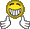# Multiplication and divisionClassicListThreaded6 messagesOpen this post in threaded view
|

## Multiplication and division

 ScreenTest has been optimized to be instantaneous, but it's very slow to replace Math. VM with my own Math. VM in drawing the roof. I think the problem should be multiplication and division, but I've found a lot of knowledge about multiplication and division on the internet. It involves masking displacement operations. Besides, are there any other better ways to make multiplication and division work faster?
Open this post in threaded view
|

## Re: Multiplication and division

 Administrator One quick thing that you can do in Math.divide is to move the recursive calling into another function that does not need to waste time checking for negative arguments ```function int divide(int x, int y) { var q; let q = Math._div(Math.abs(x), Math.abs(y)); if ((x < 0) = (y < 0)) { // sign(x) == sign(y) return q; } else { return -q; } } ```_div(int x, int y) is the normal recursive divide function. For Mult(), since you are testing the bits in order, you do not need to call a function to get the 2^n bits. Set mask to 1 and do mask = mask + mask to get the next mask value.
Open this post in threaded view
|

## Re: Multiplication and division

 This post was updated on .thank you very much, mark In accordance with your method to re realize the division and multiplication is really faster.
Open this post in threaded view
|

## Re: Multiplication and division

 In reply to this post by cadet1620 Hi, I am trying to understand how the multiplication algorithm works. I quite understood how it is working for positive numbers. It is told in the course material that the algorithm works also for the negative numbers in 2's complement. I verified it and it magically turned out to be true if we consider only the last 16 bits of the product. Although this is not a course on algorithms, I wonder how is this multiplication working out even for negative numbers. I can't figure it out or understand the reason or prove that the algorithm is valid for negative numbers also. I beg to please explain or provide a hint on why it is working for negative numbers. If you are kind enough please provide any proof or link to any source on the web that explains why it works.